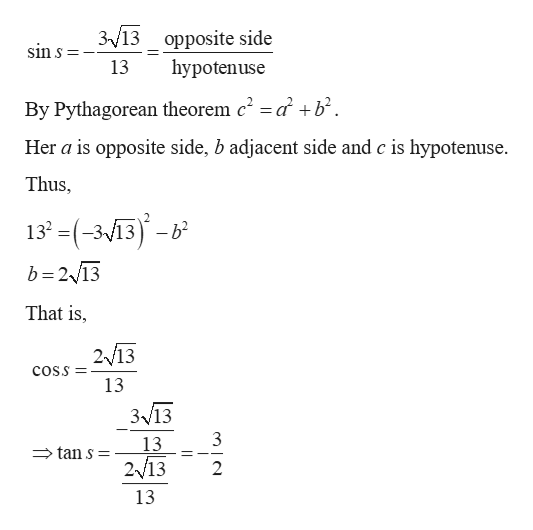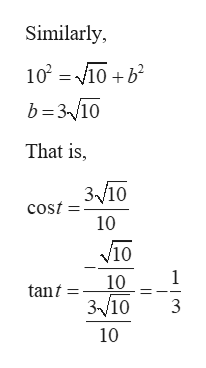# Find the exact value of the expression using the provided information.Find tan(s - t) given that sin s = - (3\sqrt(13))/(13), with s in quadrant IV, and sin t=(\sqrt(10))/(10) with t in quadrant IV

Question
9 views

Find the exact value of the expression using the provided information.

Find tan(s - t) given that sin s = - (3\sqrt(13))/(13), with s in quadrant IV, and sin t=(\sqrt(10))/(10) with t in quadrant IV

check_circle

Step 1

Given:

Step 2

Find the value of tans as follows.help_outlineImage Transcriptionclose3/13 opposite side sin s hypotenuse 13 By Pythagorean theorem c2 = a b Her a is opposite side, b adjacent side and c is hypotenuse. Thus 13 =(-313)-b b 2 13 That is, 2/13 cosS 13 3v13 3 13 tan s= 2/13 2 13 fullscreen
Step 3

Find the value of tant ...help_outlineImage TranscriptioncloseSimilarly, 102 10b b 310 That is 3/10 cost 10 V10 1 10 tant 310 3 10 fullscreen

### Want to see the full answer?

See Solution

#### Want to see this answer and more?

Solutions are written by subject experts who are available 24/7. Questions are typically answered within 1 hour.*

See Solution
*Response times may vary by subject and question.
Tagged in

### Other